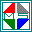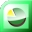Delivered by FeedBurner

 Home: \ Education \ Mathematics \ Visual Optim 2.1 \ Screenshot

Visual Optim 2.1 - Screenshot Page

Description: Visual Optim is a math program for linear and nonlinear programming... (more)

# Screenshot for Visual Optim 2.1

 Top rated in Education - Mathematics SimplexNumerica 9.4.1.0   (n/r) Best data analyzer, 2D/3D-plotting, calc and presentation program.DreamCalc DCG Graphing Calculator 4.9.2   (n/r) DreamCalc Scientific Graphing Calculator (Graphing Edition)DreamCalc DCS Scientific Calculator 4.9.2   (n/r) DreamCalc Scientific Calculator (Scientific Edition)MultiplexCalc 5.4.9   (n/r) MultiplexCalc is a multipurpose and comprehensive desktop calculator for WindowsDesktopCalc 2.1.9   (n/r) DesktopCalc is an enhanced, easy-to-use and powerful scientific calculatorCompactCalc 4.2.9   (n/r) CompactCalc is an enhanced scientific calculator for WindowsEqPlot 1.3.9   (n/r) EqPlot plots 2D graphs from complex equations.SimplexCalc 4.1.9   (n/r) SimplexCalc is a multivariable desktop calculator for Windows.CurveFitter 4.5.9   (n/r) CurveFitter performs regression analysis to estimate values of parameters.ScienCalc 1.3.9   (n/r) ScienCalc is a convenient and powerful scientific calculator.DataFitting 1.7.9   (n/r) DataFitting performs regression analysis to estimate values of parameters. InterReg 3.2.2   (n/r) Visual Interpolation and Regression analysis by simple Point-and-ClickInnoCalculator 1.1.9   (n/r) InnoCalculator is multipurpose and comprehensive desktop calculator for WindowsVisual Fitting 2.3.0   (n/r) Math tool for linear, nonlinear curve fitting and surface fitting.Visual Stats 2.0   (n/r) Statistics software for data analysis and multivariate statistical analysis.

Browse Freeware by Popular Keywords:

Browse by Title:

Browse by Developer:

Useful Resources:

Free Webmaster Software

Four Freshdevices software:

Name:

E-mail: